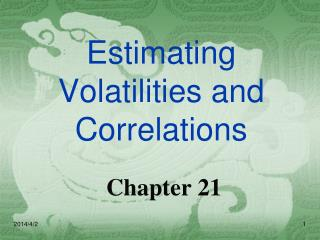DownloadDownload PresentationEstimating Volatilities and Correlations

# Estimating Volatilities and Correlations

Télécharger la présentation## Estimating Volatilities and Correlations

- - - - - - - - - - - - - - - - - - - - - - - - - - - E N D - - - - - - - - - - - - - - - - - - - - - - - - - - -
##### Presentation Transcript

1. Estimating Volatilities and Correlations Chapter 21

2. Standard Approach to Estimating Volatility • Define sn as the volatility per day on day n, as estimated at end of dayn-1 • Define Si as the value of market variable at end of day i • Define ui= ln(Si/Si-1), an unbiased estimate is

3. Simplifications Usually Made • Defineui as (Si-Si-1)/Si-1 • Assume that the mean value of ui is zero • Replace m-1 by m This gives

4. Weighting Scheme Instead of assigning equal weights to the observations we can set

5. ARCH(m) Model In an ARCH(m) model we also assign some weight to the long-run variance rate, V:

6. EWMA Model • In an exponentially weighted moving average model, the weights assigned to the u2 decline exponentially as we move back through time.

7. Especially, if

8. Attractions of EWMA • Relatively little data needs to be stored • We need only remember the current estimate of the variance rate and the most recent observation on the market variable • Tracks volatility changes • JP Morgan use l = 0.94 for daily volatility forecasting

9. GARCH (1,1) In GARCH (1,1) we assign some weight to the long-run average variance rate Since weights must sum to 1 g + a + b =1

10. GARCH (1,1) continued Setting w = gV the GARCH (1,1) model is and

11. Example • Suppose • the long-run variance rate is 0.0002 so that the long-run volatility per day is 1.4%

12. Example continued • Suppose that the current estimate of the volatility is 1.6% per day and the most recent proportional change in the market variable is 1%. • The new variance rate is The new volatility is 1.53% per day

13. GARCH (p,q)

14. Mean Reversion • The GARCH(1,1) is equivalent to a model where the variance V follows the stochastic process • GARCH(1,1) incorporates mean reversion, EWMA does not. When is negative, GARCH(1,1) is not stable, and we should use EWMA.

15. Other Models • We can design GARCH models so that the weight given to ui2 depends on whether ui is positive or negative.

16. Maximum Likelihood Methods • In maximum likelihood methods we choose parameters that maximize the likelihood of the observations occurring

17. Example 1 • We observe that a certain event happens one time in ten trials. What is our estimate of the proportion of the time, p, that it happens • The probability of the outcome is • We maximize this to obtain a maximum likelihood estimate: p=0.1

18. Example 2（Suppose the variance is constant) Estimate the variance of observations from a normal distribution with mean zero

19. Application to GARCH We choose parameters that maximize

20. Excel Application (Table 21.1, page 477) • Start with trial values of w, a, and b • Update variances • Calculate • Use solver to search for values of w, a, and b that maximize this objective function • Important note: set up spreadsheet so that you are searching for three numbers that are the same order of magnitude (See page 478)

21. Variance Targeting • One way of implementing GARCH(1,1) that increases stability is by using variance targeting • We set the long-run average volatility equal to the sample variance • Only two other parameters then have to be estimated.

22. How Good is the Model? • We compare the autocorrelation of the ui’s with the autocorrelation of the ui/si • The Ljung-Box statistic tests for autocorrelation

23. Forecasting Future Volatility A few lines of algebra shows that The variance rate for an option expiring on day m is

24. Volatility Term Structures (Table 21.4) • The GARCH (1,1) suggests that, when calculating vega, we should shift the long maturity volatilities less than the short maturity volatilities • Impact of 1% change in instantaneous volatility for Japanese yen example:

25. Correlations • Define ui=(Ui-Ui-1)/Ui-1 and vi=(Vi-Vi-1)/Vi-1 • Also su,n: daily vol of U calculated on day n-1 sv,n: daily vol of V calculated on day n-1 covn: covariance calculated on day n-1

26. Under GARCH (1,1) covn= w + aun-1vn-1+b covn-1 Correlations continued

27. Positive semi-definite Condition A variance-covariance matrix, W, is internally consistent if the positive semi-definite condition for all vectors w is satisfied.

28. Example The variance covariance matrix is not internally consistent